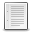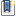File : Details

# partfrac()

Partial fraction decomposition of a rational in the C set
Details
Version
1.3.1
Author
Samuel Gougeon
Maintainer
Samuel Gougeon
Category
Supported Scilab Version
6.1
Creation Date
December 29, 2021Description
```
--> partfrac

Partial fraction decomposition of a rational in C

SYNTAX
------
partfrac    // Displays this help
I          = partfrac(r)
[I, R]      = partfrac(r)
[I, R, F]   = partfrac(r)
[I, R, F, T]= partfrac(r)

PARAMETERS
----------
r : Single rational = polynomial fraction with real or complex coefficients.
I : Integer part = non-fractional part of f (single polynomial).
R : Remainder of r: rational with degree(numerator) < degree(denominator)

F : (3xN) matrix describing the N terms of the r's decomposition:
F(1,:): real or complex coefficient of numerators.
F(2,:): real or complex value of the considered poles.
F(3,:): integer>0: powers m of the denominators (x-pole)^m
F is such that sum( F(1,:)./ ((x-F(2,:)).^F(3,:)) ) - r == 0.

T : 3-rows column of Texts. write(%io(2),t) displays i+d in a comprehensive
way. The format of coefficients is set with format().

DESCRIPTION
-----------
From a single polynomial fraction r = p/q with coprime polynomials p and q,
partfrac(f) extracts
* the non fractional part I of f: polynomial such that
0 <= degree(I) <= degree(p) - degree(q): I = p - modulo(p,q)
* the fractional part of r, or remainder R of p/q : R = modulo(p,q)/q
such that degree(r.num))< degree(q) and  r = I + R
* the rational decomposition of R, aka partial fraction decomposition of r:
The 'vector' of elementary rationals c/(x-pole)^m where c and poles are
decimal or complex numbers and m are the multiplicities of poles:
c = F(1,:), pole = F(2,:), m = d(3,:)

In addition, partfrac() may return as text the literal expression T of the
whole decomposition of r. This form shows the factorized forms of denominators
where poles that are multiple appear with their powers. write(%io(2), T) may
be used to display it.

DEPENDENCY: If F or/and T is expected, polyroots() is required (=>See also)

NOTE: pfss(r) yields a list mixing the non-fractional part (as last element)
with the decomposition, and where terms of the decomposition are not always
elementary: their denominator may be of order 2 even for real poles. See the
example.

REFERENCE
---------
Comments, scoring and bug reports are welcome on
http://fileexchange.scilab.org/toolboxes/451000#new_comment

--------
pfss      : Partial (uncomplete) fraction decomposition of a linear system
modulo    : Remainder after polynomial division
polyroots : Multiplicities and values of polynomial multiple roots:
http://fileexchange.scilab.org/toolboxes/362000
pdiv_inc  : Polynomial division with increasing terms powers:
http://fileexchange.scilab.org/toolboxes/449000

EXAMPLE
-------
x = poly(0,"x");
r = (3-x+x^2-4*x^3+2*x^4) / ((x-1)*(x-2)^2)
[I, R, F, T] = partfrac(r);
I, R, F, T
D = F(1,:)./((x-F(2,:)).^F(3,:))
clean(R-sum(D))
// Comparison with pfss():
list2vec(pfss(r)).'

RESULTS
-------

-->  x = poly(0,"x");

-->  r = (3-x+x^2-4*x^3+2*x^4) / ((x-1)*(x-2)^2)
r  =

3 -x +x² -4x³ +2x⁴
------------------
-4 +8x -5x² +x³

-->  [I, R, F, T] = partfrac(r);

-->  I, R, F, T
I  =
6 +2x

R  =
27 -41x +15x²
---------------
-4 +8x -5x² +x³

F  =
14.   5.   1.
2.    2.   1.
1.    2.   1.

T  =
"         14       5        1  "
"6 +2x + ---- + -------- + ----"
"        -2+x   (-2+x)^2   -1+x"

-->  D = F(1,:)./((x-F(2,:)).^F(3,:))
D  =

14        5        1
-----  ---------  -----
-2 +x  4 -4x +x²  -1 +x

-->  clean(R-sum(D))
ans  =
0
-
1

-->  // Comparison with pfss():

-->  list2vec(pfss(r)).'
ans  =

-23 +14x     1    6 +2x
---------  -----  -----
4 -4x +x²  -1 +x    1
```Files (1)OS-independent binary for Scilab .x
```CHANGES:
* Text output formating updated for Scilab 6.1
Therefore, this version can't be used with Scilab < 6.1
* When the variable name contains "%", a printing error occurred:
Fixed.
* polyroots() is included/appended.

```News (0)Comments (0)Leave a comment
Login withEmail notifications